# WBJEE 2019 Physics Paper with Solutions

WBJEE 2019 Physics question paper and solutions are available here. WBJEE is an examination conducted for admission into B.Tech and B.Pharma courses in different colleges in the state of West Bengal. The questions have been solved by our subject experts and they have come with easy to understand solutions that will further help students to get a clear knowledge of the concepts. The question paper can be downloaded in a pdf format and used for offline practice. Candidates can boost their preparation for the entrance examination by practising and solving the WBJEE 2019 question paper.

### WBJEE 2019 - Physics

Question 1: A point object is placed on the axis of a thin convex lens of focal length 0.05 m at a distance of 0.2m from the lens and its image is formed on the axis. If the object is now made to oscillate along the axis with small amplitude of A cm, then what is the amplitude of oscillation of the image? (you may assume (1/(1+x) ≈ 1- x, where x≪1)

1. a. (4A/9) x 10-2 m
2. b. (5A/9) x 10-2 m
3. c. (A/3) x 10-2 m
4. d. (A/9) x 10-2 m

Solution: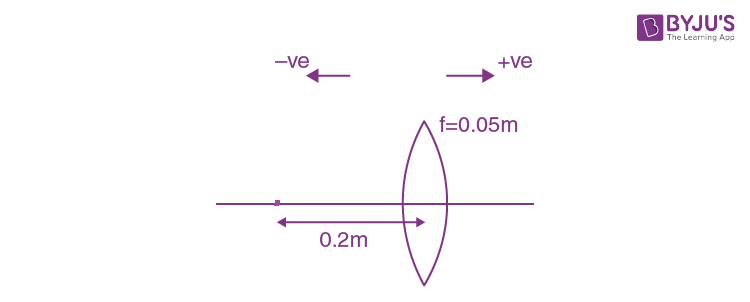u = -0.2 m

f = 0.05 m

By using lens formula,

$$\frac{1}{v}=\frac{1}{f}+\frac{1}{u}$$

$$\frac{1}{v}=\frac{100}{5}-\frac{10}{2}$$

$$\frac{1}{v}=\frac{1}{15}m$$

Let Aimage be the amplitude of the image.

$$\Rightarrow -\frac{dv}{v^{2}}=-\frac{du}{u^{2}}$$

$$\Rightarrow dv=du\times \frac{v^{2}}{u^{2}}$$

$$A_{image}=A.\frac{v^{2}}{u^{2}}$$

Substituting values,

$$A_{image}=A.\frac{25}{225}$$

$$A_{image}=\frac{A}{9}\times 10^{-2}m$$

Question 2: In Young's double slit experiment, d is the separation between the slits and D is the distance between the plane of the slits and the screen. If D is increased by 0.5% and d is decreased by 0.3% then, for a given light of a certain wavelength, which one of the following options is correct?

"It is the fringe width ................."

1. a. increases by 0.8%
2. b. decreases by 0.8%
3. c. increases by 0.2%
4. d. decreases by 0.2%

Solution:

The fringe width is given by: β= λD/d

Where, D → distance of screen from slits

d → width of the slit

Now,

$$\frac{\Delta \beta }{\beta }\times 100=\frac{\Delta D}{D}\times 100-\frac{\Delta d}{d}\times 100$$

= 0.5 – (–0.3) = 0.8%

Hence, the fringe width increases by 0.8%.

Question 3: When the frequency of the light used is changed from 4 × 1014 s–1to 5 × 1014 s–1, the angular width of the principal (central) maximum in a single slit Fraunhofer diffraction pattern changes by 0.6 radian. What is the width of the slit (assume that the experiment is performed in a vacuum)

1. a. 1.5 × 10–7 m
2. b. 3 × 10–7m
3. c. 5 × 10–7m
4. d. 6 × 10–7m

Solution:

Change in angular width, Δ θ= 0.6 rad.

Wavelength of light is given by,

$$\lambda =\frac{c}{\nu }$$

c→Speed of light

ν→Frequency of light

Change in wavelength;

$$\Delta \lambda =\frac{c}{\nu_{1} }-\frac{c}{\nu_{2} }$$

$$\Delta \lambda =3\times 10^{8}\left [ \frac{1}{4\times 10^{14} }-\frac{1}{5\times 10^{14} } \right ]$$

= 1.5 x 10-7 m

Angular width of central maxima is,

$$\theta =\frac{2\lambda }{d}$$

$$\Delta \theta =\frac{2\Delta \lambda }{d}$$

$$d=\frac{2\Delta \lambda }{\Delta \theta}$$

$$d=\frac{2\times 1.5\times 10^{-7} }{0.6}$$

$$d= 5\times 10^{-7}m$$

Question 4: A ray of light is reflected by a plane mirror

$$\hat{e_{0}}$$
,
$$\hat{e}$$
and
$$\hat{n}$$
be the unit vectors along the incident ray, reflected ray and the normal to the reflecting surface respectively. Which of the following gives an expression for
$$\hat{e}[\latex]?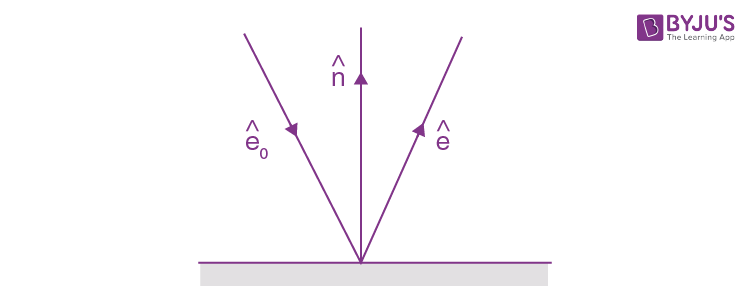1. a. \(\hat{e_{0}}+2(\hat{e_{0}}.\hat{n})\hat{n}0$$
2. b.
$$\hat{e_{0}}-2(\hat{e_{0}}.\hat{n})\hat{n}$$
3. c.
$$\hat{e_{0}}-(\hat{e_{0}}.\hat{n})\hat{n}$$
4. d.
$$\hat{e_{0}}+(\hat{e_{0}}.\hat{n})\hat{n}$$

Solution: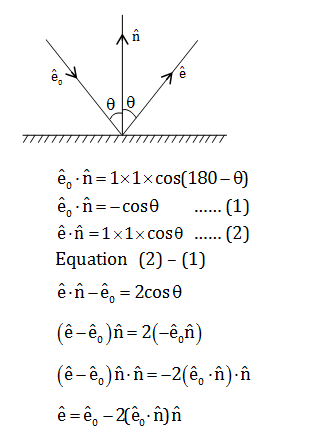Question 5: A parent nucleus X undergoes α-decay with a half-life of 75000 years. The daughter nucleus Y undergoes β-decay with a half-life of 9 months. In a particular sample, it is found that the rate of emission of β-particles is nearly constant (over several months) at 107/hour. What will be the number of α-particles emitted in an hour?

1. a. 102
2. b. 107
3. c. 1012
4. d. 1014

Solution:

When emission of β- particles is nearly constant then the number of nucleus of y at that duration is constant.

So,

$$\frac{dN_{y}}{dt}=0$$

Ny →Number of daughter nuclei at timed.

$$\Rightarrow \lambda _{x}N_{x}-\lambda _{y}N_{y}=\frac{dN_{y}}{dt}$$

λxNx rate of α particle emission

λyNy rate of β particle emission

λxNx – λyNy = 0

λxNx = λyNy = 107/hours

Question 6: A proton and an electron initially at rest are accelerated by the same potential difference. Assuming that a proton is 2000 times heavier than an electron, what will be the relation between the de Broglie wavelength of the proton (λp) and that of electron (λc)?

1. a.
$$\lambda _{p}=2000\lambda _{e}$$
2. b.
$$\lambda _{p}=\frac{\lambda _{e}}{2000}$$
3. c.
$$\lambda _{p}=20\sqrt{5}\lambda _{e}$$
4. d.
$$\lambda _{p}=\frac{\lambda _{e}}{20\sqrt{5}}$$

Solution:

De Broglie wavelength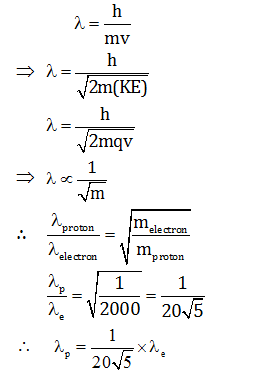Question 7: To which of the following, the angular velocity of the electron in the nth Bohr orbit is proportional?

1. a. n2
2. b. (1/n2)
3. c. (1/n3/2)
4. d. (1/n3 )

Solution:

Angular momentum:

$$L=mr^{2}\omega =\frac{nh}{2\pi }$$

$$\omega \propto \frac{n}{r^{2} }$$

$$\omega \propto \frac{n}{(r_{0}n^{2})^{2}}$$

$$\Rightarrow \omega \propto \frac{1}{n^{3}}$$

Question 8: In the circuit shown, what will be the current through the 6V zener?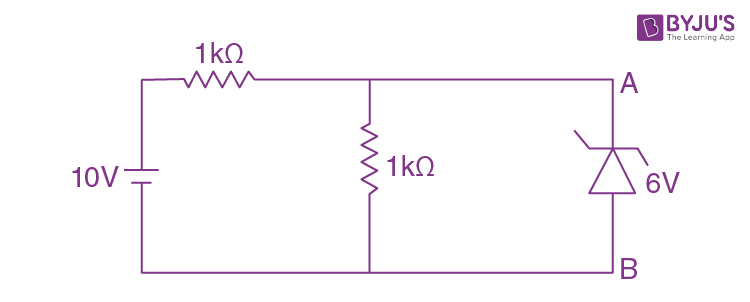1. a. 6mA, from A to B
2. b. 2mA, from A to B
3. c. 2 mA, from B to A
4. d. zero

Solution:

The voltage across Zener diode will be,

$$V=10\times \left [ \frac{1}{1+1} \right ]\Rightarrow V=5V$$

As, the breakdown voltage of Zener diode is 6V and the potential difference across the diode is only 5V, therefore it will not conduct.

∴ No current will flow

Question 9: Each of the two inputs A and B can assume values either 0 or 1. Then which of the following will be equal to

$$\bar{A}.\bar{B}$$
?

1. a. A + B
2. b.
$$\overline{A+B}$$
3. c.
$$\overline{A.B}$$
4. d.
$$\bar{A}+\bar{B}$$

Solution:

By using Demorgen's law

$$\bar{A}.\bar{B}=\overline{A+B}$$

Question 10: The correct dimensional formula for impulse is given by

1. a. ML2T–2
2. b. MLT–1
3. c. ML2T–1
4. d. MLT–2

Solution:

Impulse = charge in momentum

I = ΔP

$$\Rightarrow \left [ I \right ]=\left [ \Delta P \right ]=\left [ MLT^{-1} \right ]$$

Question 11: The density of the material of a cube can be estimated by measuring its mass and the length of one of its sides. If the maximum error in the measurement of mass and length are 0.3% and 0.2% respectively, the maximum error in the estimation of the density of the cube is approximately

1. a.1.1%
2. b.0.5%
3. c.0.9%
4. d.0.7%

Solution:

The density of the cube is

$$\rho =\frac{M}{V}$$

M → mass of cube

V →Volume of cube

Also, V = L3

$$\frac{\Delta V}{V}=\frac{3\Delta L}{L}$$

∴Maximum error in density

$$\left(\frac{\Delta \rho}{\rho}\right)_{\max }=\left(\frac{\Delta {m}}{m}\right)+\left(\frac{\Delta {V}}{{V}}\right)$$

$$\left(\frac{\Delta \rho}{\rho}\right)_{\max }=\left(\frac{\Delta {m}}{m}\right)+3\left(\frac{\Delta {L}}{{L}}\right)$$

$$\left(\frac{\Delta \rho}{\rho}\right)_{\max }\times 100=[0.3+3(0.2))]%$$

= 0.9%

Question 12: Two weights of the mass m1and m2(> m1) are joined by an inextensible string of negligible mass passing over a fixed frictionless pulley. The magnitude of the acceleration of the loads is

1. a. g
2. b.
$$\frac{m_{2}-m_{1}}{m_{2}}g$$
3. c.
$$\frac{m_{1}}{m_{2}+m_{1}}g$$
4. d.
$$\frac{m_{2}- m_{1}}{m_{2}+m_{1}}g$$

Solution: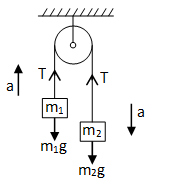m2g - T= m2a …… (1)

T – m1g = m1a …… (2)

On solving equation (1) & (2)

$$a =\left [ \frac{m_{2}-m_{1}}{m_{2}+m_{1}} \right ]g$$

Question 13: Body starts from rest, under the action of an engine working at a constant power and moves along a straight line. The displacement S is given as a function of time (t) as

1. a. S = at + bt2, a, b are constants
2. b. S = bt2, b is a constant
3. c. S = at3/2 ,a is a constant
4. d. S = at, a is a constant

Solution:

P = W/t

P →Power (Constant)

W→ Work done

t → time taken

The work done is equal to change in kinetic energy

$$P=\frac{\Delta KE}{\Delta t}=\frac{1/2mv^{2}}{t}=\frac{mv^{2}}{2t}$$

$$\Rightarrow V\propto \sqrt{t}$$

$$\frac{ds}{dt}\propto \sqrt{t}$$

$$S\propto t^{3/2}$$

Question 14: Two particles are simultaneously projected in the horizontal direction from a point P at a certain height. The initial velocities of the particles are oppositely directed to each other and have magnitude v each. The separation between the particles at a time when their position vectors (drawn from the point P) are mutually perpendicular, is

1. a. v2/2g
2. b. v2/g
3. c. 4v2/g
4. d. 2v2/g

Solution:

Let

$$\vec{r_{1}}$$
and
$$\vec{r_{2}}$$
be the position vector of two particles which are given by,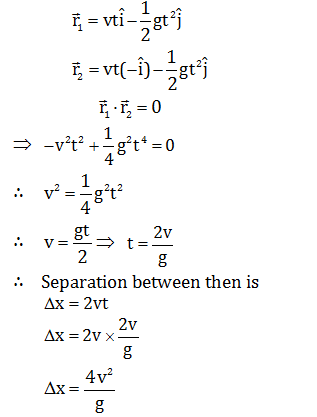Question 15: Assume that the earth moves around the sun in a circular orbit of radius R and there exists a planet which also moves around the sun in a circular orbit with an angular speed twice as large as that of the earth. The radius of the orbit of the planet is

1. a. 2-2/3R
2. b. 22/3R
3. c. 2–1/3R
4. d. R/√2

Solution:

$$T^{2}\propto r^{3}$$

T →Time period

r →radius of orbit

$$\Rightarrow \frac{r_{E}}{r_{p}}=\left [ \frac{T_{E}}{T_{p}} \right ]^{2/3}$$

$$\Rightarrow \frac{r_{E}}{r_{p}}=\left [ \frac{\omega _{E}}{\omega _{p}} \right ]^{2/3}$$

(since,

$$T\propto \omega$$
)

$$\Rightarrow \frac{R}{r_{p}}=(2)^{\frac{2}{3}}$$

$${r_{p}}=(2)^{-\frac{2}{3}}R$$

Question 16: A compressive force is applied to a uniform rod of rectangular cross-section so that its length decreases by 1%. If the Poisson's ratio for the material of the rod be 0.2, which of the following statements is correct?

1. a. decreases by 1%
2. b. decreases by 0.8%
3. c. increases by 0.6%
4. d. increases by 0.2%

Solution:

V = Al

V = abl

A → area of cross-section of the rectangle of sides a and b.

l→ length

Poison's ratio is given by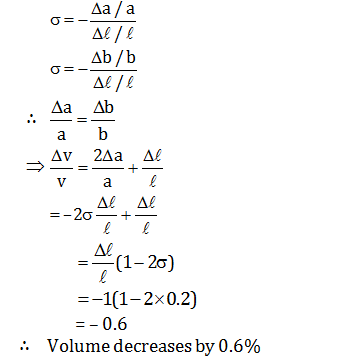Question 17: A small spherical body of radius r and density ρ moves with the terminal velocity v in a fluid of coefficient of viscosity η and density σ. What will be the net force on the body?

1. a.
$$\frac{4\pi }{3}r^{3}(\rho -\sigma )g$$
2. b. 6πηrv
3. c. Zero
4. d. Infinity

Solution:

Body is moving with terminal velocity, it means net force acting on the body is zero.

Question 18: Two black bodies A and B have equal surface areas and are maintained at temperatures 27°C and 177°Crespectively. What will be the ratio of the thermal energy radiated per second by A to that by B?

1. a. 4: 9
2. b. 2: 3
3. c. 16: 81
4. d. 27: 177

Solution:

$$Q=\sigma T^{4}$$

$$\frac{Q_{1}}{Q_{2}}=\left [ \frac{T_{1}}{T_{2}} \right ]^{4}$$

$$=\left [ \frac{273+27}{273+177} \right ]^{4}$$

$$=\left [ \frac{300}{450} \right ]^{4}$$

= 16/81

Question 19: What will be the molar specific heat at constant volume of an ideal gas consisting of rigid diatomic molecules?

1. a. (3/2)R
2. b. (5/2)R
3. c. R
4. d. 3R

Solution:

Molar heat capacity of a gas; Cv= fR/2

R →Universal gas constant

f →Degree of freedom

for diatomic gas; f = 5

∴ Cv = 5R/2

Question 20: Consider the given diagram. An ideal gas is contained in a chamber (left) of volume V and is at an absolute temperature T. It is allowed to rush freely into the right chamber of volume V which is initially vacuum. The whole system is thermally isolated. What will be the final temperature of the system after the equilibrium has been attained?1. a. T
2. b. T/2
3. c. 2T
4. d. T/4

Solution:

When there is free expansion.

Δu = 0

As Δu =nCv ΔT

ΔT = 0

Ti=Tf=T

Question 21: Five identical capacitors of capacitance 20μF each are connected to a battery of 150V, in combinations shown in the diagram. What is the total amount of charge stored?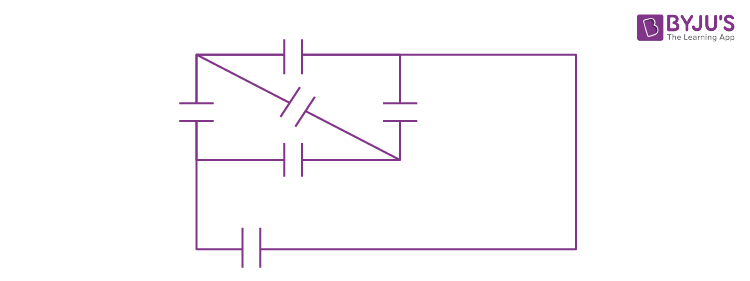1. a. 15 × 10–3C
2. b. 12 × 10–3C
3. c. 10 × 10–3C
4. d. 3 × 10–3C

Solution:

Given condition is of balanced wheat stone bridge.

∴ Ceq = 20μF

∴ Amount of stored charge is

Q = Ceq. V

= 20×10-6×150

= 3×10-3C

Question 22: Eleven equal point charges, all of them having a charge +Q, are placed at all the hour positions of a circular clock of radius r, except at the 10 hour position. What is the electric field strength at the centre of the clock?

1. a.
$$\frac{Q}{4\pi \varepsilon _{0}r^{2}}$$
from the centre towards the mark 10
2. b.
$$\frac{Q}{4\pi \varepsilon _{0}r^{2}}$$
from the mark 10 towards the centre
3. c.
$$\frac{Q}{4\pi \varepsilon _{0}r^{2}}$$
from the centre towards the mark 6
4. d. Zero

Solution:

Had all the hour positions were occupied by +Q charges, then the net electric field strength at the centre of the clock would have been zero, as due to symmetry of charge positions they would have cancelled electric field due to each other.

∴ E1 + E2 +............................. E11 + E12 = 0

∴ E1 + E2 +............................. E11 + E12 = –E10

∴ The electric field due to point charge at 10th-hour positions will be

$$\frac{Q}{4\pi \varepsilon _{0}r^{2}}$$
and the direction is from the centre towards the mark 10

Question 23: A negative charge is placed at the midpoint between two fixed equal positive charges, separated by a distance 2d. If the negative charge is given a small displacement x (x << d) perpendicular to the line joining the positive charges, how the force (F) developed on it will approximately depend on x?

1. a. F ∝ x
2. b. F ∝ (1/x)
3. c. F ∝ x2
4. d. F ∝ (1/x2)

Solution: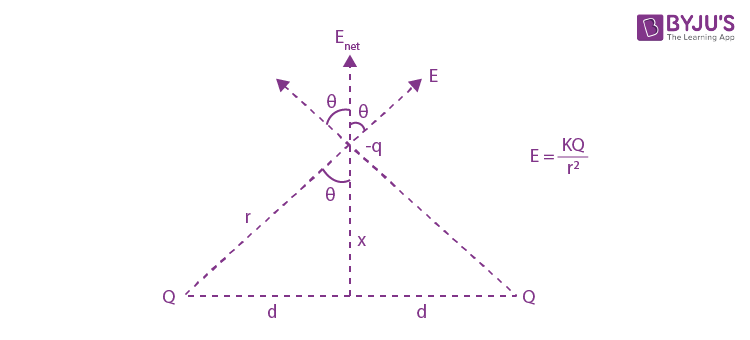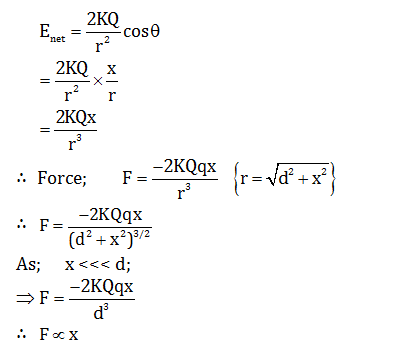Question 24: To which of the following quantities, the radius of the circular path of a charged particle moving at right angles to a uniform magnetic field is directly proportional?

1. a. energy of the particle
2. b. magnetic field
3. c. charge of the particle
4. d. Momentum of the particle

Solution:

The radius R of the circular path of a charged particle q in uniform magnetic field B is given by

∴ R = (mv/qB) = (p/qB) [since, p = mv]

m→ mass of the charged particle

v→ velocity of charged particle

p→ momentum of charged particle

∴ R ∝p

Question 25: An electric current 'I' enters and leaves a uniform circular wire of radius r through diametrically opposite points. A particle carrying a charge q moves along the axis of the circular wire with speed v. What is the magnetic force experienced by the particle when it passes through the centre of the circle?

1. a.
$$qv\frac{\mu _{0}i}{a}$$
2. b.
$$qv\frac{\mu _{0}i}{2a}$$
3. c.
$$qv\frac{\mu _{0}i}{2\pi a}$$
4. d. zero

Solution: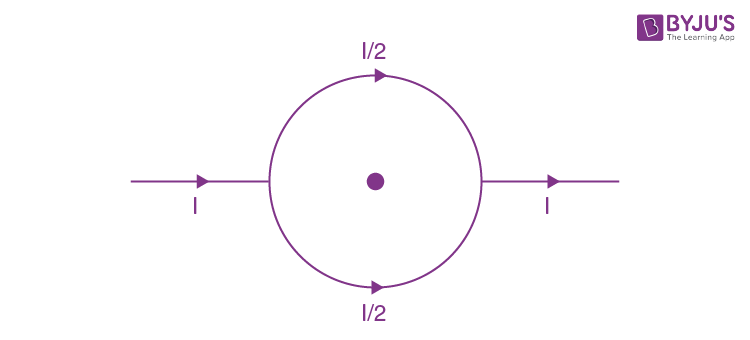Net magnetic field at the centre will be zero, as the magnitude of the magnetic field due to each half-circle will be same but opposite in direction.

Now,

$$\vec{F}=q(\vec{V\times \vec{B}})$$

$$\vec{F}=q(\vec{V\times 0})$$

$$\vec{F}=0$$

Question 26: A current 'I' is flowing along an infinite, straight wire, in the positive Z-direction and the same current is flowing along a similar parallel wire 5 m apart, in the negative Z-direction. A point P is at a perpendicular distance 3 m from the first wire and 4 m from the second. What will be the magnitude of the magnetic field Bat P?

1. a.
$$\frac{5}{12}(\mu _{0}I)$$
2. b.
$$\frac{7}{24}(\mu _{0}I)$$
3. c.
$$\frac{5}{24}(\mu _{0}I)$$
4. d.
$$\frac{25}{288}(\mu _{0}I)$$

Solution:

Question 27: A square conducting loop is placed near an infinitely long current-carrying wire with one edge parallel to the wire as shown in the figure. If the current in the straight wire is suddenly halved. Which of the following statements will be true?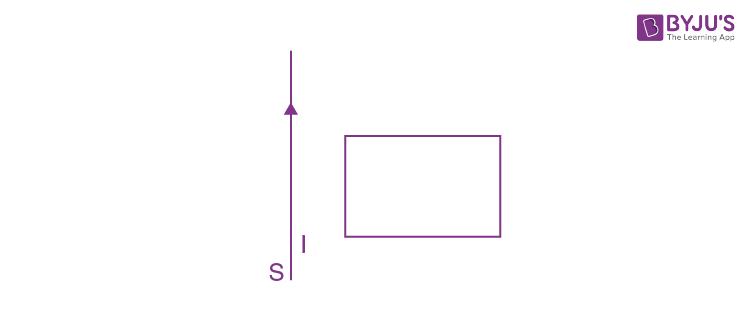''The loop will ……… ''

1. a. stay stationary.
2. b. move towards the wire.
3. c. move away from the wire.
4. d. move parallel to the wire.

Solution:Induced current in the loop will be in clockwise direction, so it will move towards the wire.

Question 28: What is the current I shown in the given circuit?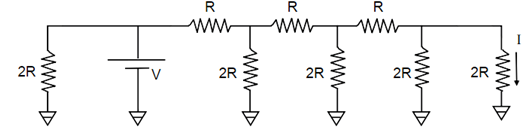1. a. V/2R
2. b. V/R
3. c. V/16R
4. d. V/8R

Solution:

The given circuit can be redrawn as: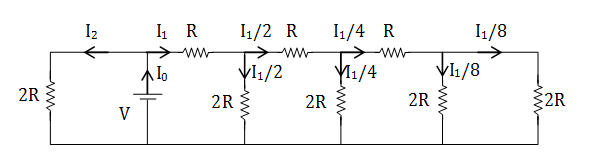Above circuit can be redrawn as: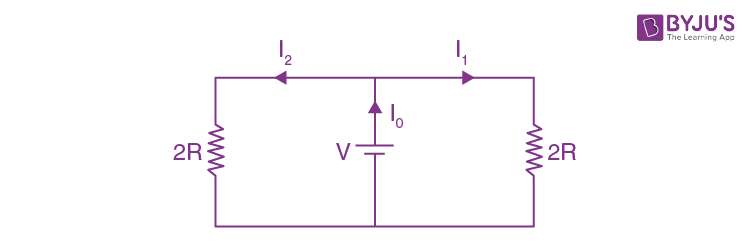I1=V/2R

(I1/8) = (V/16R)

Question 29: When the value of R in the balanced Wheatstone bridge, shown in the figure, is increased from 5 Ω to 7 Ω, the value of S has to be increased by 3 Ω in order to maintain the balance. What is the initial value of S?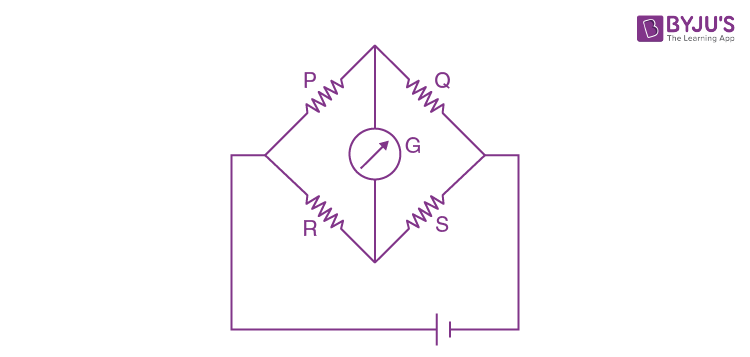1. a. 2.5 Ω
2. b. 3 Ω
3. c. 5 Ω
4. d. 7.5 Ω

Solution:

For a balanced wheat stone bridge

$$\frac{P}{Q}=\frac{R}{S}$$

As the values of P& Q are kept constant so, their ratio will remain constant

$$\frac{5}{S}=\frac{7}{S+3}$$

∴ 5S + 15 = 7S

∴ 2S = 15

∴S = 7.5 Ω

Question 30: When a 60 mH inductor and a resistor are connected in series with an AC voltage source, the voltage leads the current by 60°. If the inductor is replaced by a 0.5 μF capacitor, the voltage lags behind the current by 30°. What is the frequency of the AC supply?

1. a.
$$\frac{1}{2\pi }\times 10^{4}Hz$$
2. b.
$$\frac{1}{\pi }\times 10^{4}Hz$$
3. c.
$$\frac{3}{2\pi }\times 10^{4}Hz$$
4. d.
$$\frac{1}{2\pi }\times 10^{4}Hz$$

Solution:

When the inductor is connected with resistance,

$$tan60^{0}=\frac{\omega L}{R}$$
……(1)

When the capacitor is connected with resistance,

$$tan60^{0}=\frac{\frac{1}{\omega c}}{R}=\frac{1}{\omega cR}$$
……(2)

Dividing equation (1) by (2)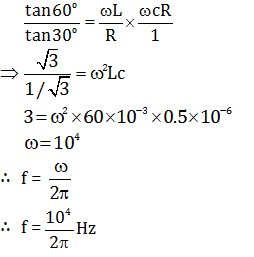Question 31: A parallel plate capacitor in series with a resistance of 100 Ω, an inductor of 20 mH and an AC voltage source of variable frequency shows a resonance at a frequency of (1250/ π) Hz. If this capacitor is charged by a DC voltage source to a voltage 25V, what amount of charge will be stored in each plate of the capacitor?

1. a. 0.2 μC
2. b. 2 mC
3. c. 0.2 mC
4. d. 0.2 C

Solution:

Angular frequency at resonance is given by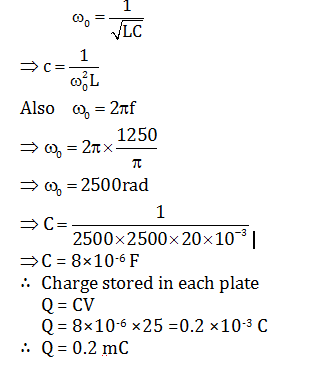Question 32: A capacitor of capacitance C is connected in series with a resistance R and a DC source of emf E through a key. The capacitor starts charging when the key is closed. By the time the capacitor has been fully charged, what amount of energy is dissipated in the resistance R?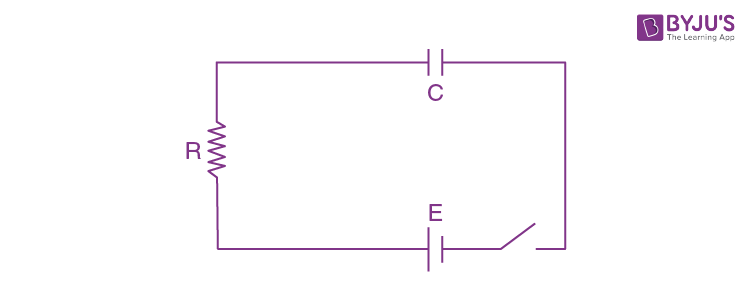1. a. (1/2) CE2
2. b. 0
3. c. CE2
4. d. E2/R

Solution:

Energy stored in capacitor = (1/2) CE2

Energy supplied by the source of emf = CE2

∴ Energy dissipated in resistance = CE2-(1/2) CE2

= (1/2) CE2

Question 33: A horizontal fire hose with a nozzle of cross-sectional area

$$\frac{5}{\sqrt{21}}\times 10^{-3}m^{2}$$
delivers a cubic metre of water in 10s. What will be the maximum possible increase in the temperature of water while it hits a rigid wall (neglecting the effect of gravity)?

1. a. 1°C
2. b. 0.1°C
3. c. 10°C
4. d. 0.01°C

Solution:

(dV/dt) = A.v

A →Area of cross section of the nozzle

V →Volume

v→ velocity

$$\frac{1}{\sqrt{10}}=\frac{5}{\sqrt{21}}\times 10^{-3}V$$

$$V=20\sqrt{21}m/s$$

Now,

$$\frac{1}{2}mv^{2}=ms\Delta T$$

$$\Delta T=\frac{V^{2}}{2S}$$

S→4.2 x 103 J/kg

ΔT = 10C

Question 34: Two identical blocks of ice move in opposite directions with equal speed and collide with each other. What will be the minimum speed required to make both the blocks melt completely, if the initial temperatures of the blocks were –8°C each?

1. a. 840 ms–1
2. b. 420 ms–1
3. c. 8.4 ms–1
4. d. 84 ms–1

Solution:

By energy conservation

$$2\times \frac{1}{2}mu^{2}=2\times [ms\Delta T+mL]$$

$$\Rightarrow u^{2}=2[S\Delta T+L]$$

S→ Specific heat capacity of ice = 2100 J/kgK

L→ Latent heat of fusion of ice = 336 KJ/kg

U2= 2[2100 x (8)+336000]

∴u = 840 m/s

Question 35: A particle with charge q moves with a velocity v in a direction perpendicular to the directions of uniform electric and magnetic fields, E and B respectively, which are mutually perpendicular to each other. Which one of the following gives the condition for which the particle moves undeflected in its original trajectory?

1. a. V= E/B
2. b. V = B/E
3. c.
$$v=\sqrt{\frac{E}{B}}$$
4. d.
$$v=q\frac{B}{E}$$

Solution:

Let the charge on the particle is q.

Force on the particle due to electric field,

FE = qE

And force on the particle due to the magnetic field,

FB = qVB

⇒As the particle moved undeflected in its original trajectory then

qE = qVB

Therefore, V= E/B

Question 36: A metallic loop is placed in a uniform magnetic field B with the plane of the loop perpendicular to B. Under which conditions(s) given below an emf will be induced in the loop?

"If the loop is ............."

1. a. Moved along the direction of B
2. b. Squeezed to a smaller area.
3. c. Rotated about its axis
4. d. Rotated about one of its diameters.

Solution:

Emf is induced when there is a change in flux with respect to time. Out of the given options only in case of option b & d flux will charge.

Question 37: Electrons are emitted with kinetic energy T from a metal plate by irradiation of light of intensity J and frequency v. Then which of the following will be true?

1. a. T ∝J
2. b. T linearly increasing with v
3. c. T ∝ time of irradiation
4. d. Number of electrons emitted ∝ J

Solution:

Kinetic energy of photoelectrons emitted.

T = hν- Φ

⇒ T ∝ν

Also, J = (dN/dt)( hν)

⇒ J∝ν

⇒T∝ J

Question 38: The initial pressure and volume of a given mass of an ideal gas (with Cp/Cv= γ), taken in a cylinder fitted with a piston, are P0 and V0 respectively. At this stage, the gas has the same temperature as that of the surrounding medium which is T0. It is adiabatically compressed to a volume equal to V0/2. Subsequently, the gas is allowed to come to thermal equilibrium with the surroundings. What is the heat released to the surroundings?

1. a. 0
2. b.
$$(2^{\gamma-1}-1)\frac{P_{0}V_{0}}{r-1}$$
3. c.
$$\gamma P_{0}V_{0}In2$$
4. d.
$$\frac{P_{0}V_{0}}{2(\gamma -1)}$$

Solution: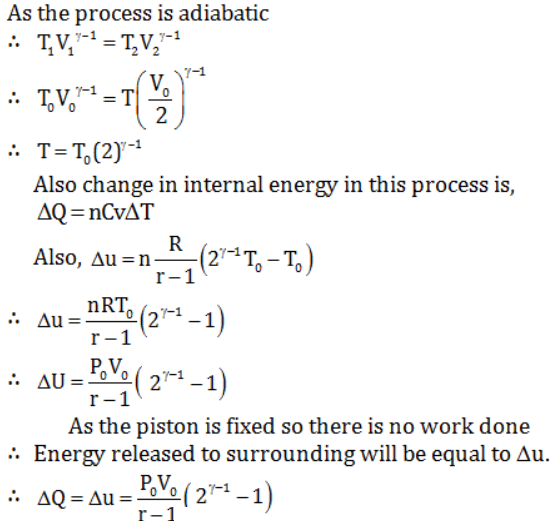Question 39: A projectile thrown with an initial velocity of 10ms–1 at an angle α with the horizontal, has a range of 5m.Taking g = 10ms–2 and neglecting air resistance, what will be the estimated value of α?

1. a. 15°
2. b. 30°
3. c. 45°
4. d. 75°

Solution:

Range,

$$R=\frac{u^{2}sin2\alpha }{g}$$

$$5=\frac{100\times sin2\alpha }{10}$$

$$sin(2\alpha )=\frac{1}{2}$$

$$2\alpha = 30^{0}$$

Therefore,

$$\alpha = 15^{0}$$

Also, sin(2α) = (1/2)

⇒ 2 α =1500

Therefore, α = 750

Question 40: In the circuit shown in the figure all the resistances are identical and each has the value r Ω. The equivalent resistance of the combination between the points A and B will remain unchanged even when the following pairs of point A and B will remain unchanged even when the following pairs of point s marked in the figure are connected through a resistance R.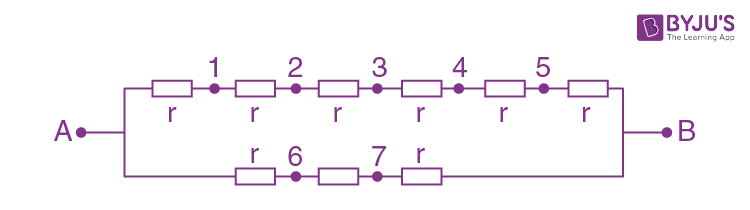1. a. 2 and 6
2. b. 3 and 6
3. c. 4 and 7
4. d. 4 and 6

Solution:

For balanced Wheat stone bridge ratio of adjacent side should be same

### WBJEE 2019 Physics Question Paper with Solutions